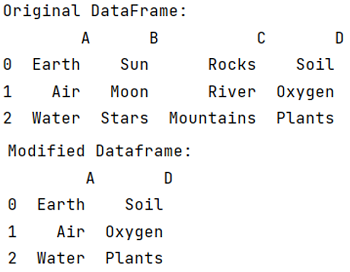# How to delete all columns in DataFrame except certain ones?

Given a Pandas DataFrame, we have to delete all columns in it except certain ones. By Pranit Sharma Last updated : September 24, 2023

## Deleting all columns in DataFrame except certain ones

For this purpose, we will use pandas.DataFrame.drop() method inside which we will pass the column difference i.e., the list of those columns which we do not want to delete. The DataFrame.drop() Method method is used to remove a specified row or column from the pandas DataFrame. Since rows and columns are based on index and axis values respectively, by passing the index or axis value inside DataFrame.drop() method we can delete that particular row or column. Below is the syntax:

### Syntax

```DataFrame.drop(
labels=None,
axis=0,
index=None,
columns=None,
level=None,
inplace=False,
errors='raise'
)
```
Note

To work with pandas, we need to import pandas package first, below is the syntax:

```import pandas as pd
```

Let us understand with the help of an example,

## Python program to delete all columns in DataFrame except certain ones

```# Importing pandas package
import pandas as pd

# Importing numpy package
import numpy as np

# Creating a dictionary
d = {
'A':['Earth','Air','Water'],
'B':['Sun','Moon','Stars'],
'C':['Rocks','River','Mountains'],
'D':['Soil','Oxygen','Plants']
}

# Creating a DataFrame
df = pd.DataFrame(d)

# Display original DataFrame
print("Original DataFrame:\n",df,"\n")

# Deleting all columns except the specified columns
df.drop(df.columns.difference(['A','D']), 1, inplace=True)

# Display result
print("Modified Dataframe:\n",df)
```

### Output

The output of the above program is: